|

# 亲自然·轻生活 仁豪•摩迪卡梦之楠家具评测

轻生活，将一切简化到最简单、最纯净的境界，抛弃掉累赘和负担，亲近自然，轻简有度，还原生活的本真。梦想中的轻生活，有璀璨的阳光，有木质的清香，有闲暇的时光，还有专属的天地。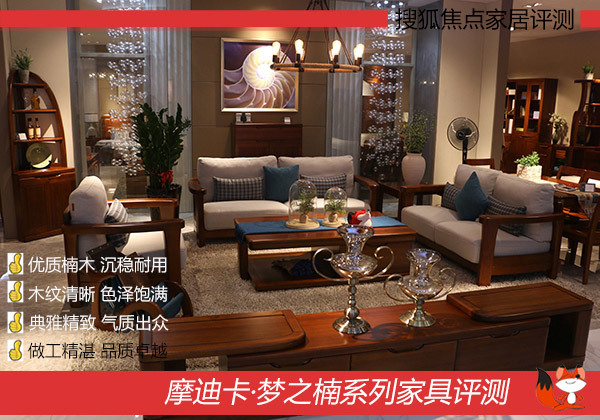而摩迪卡梦之楠，不断追求极致美好的家居风格，让梦想的轻生活成为现实。今天，搜狐焦点家居就对梦之楠系列家具进行细致评测，赶紧跟着小狐一一品鉴吧。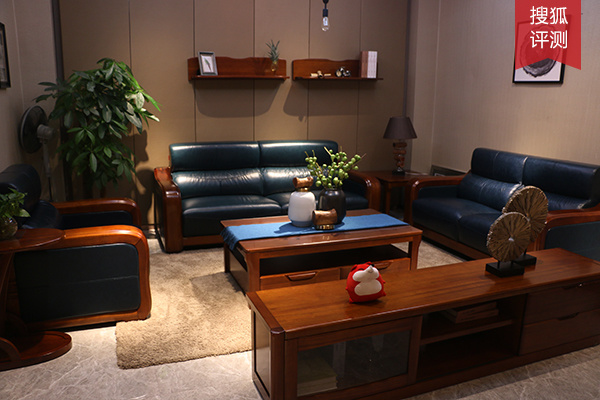楠木的棕，结合皮革深邃的蓝，打破了单调的氛围，为空间增加了质感与清新。看似简单的沙发、茶几，却拼凑出了自然典雅的客厅空间，给你安稳自在的轻生活。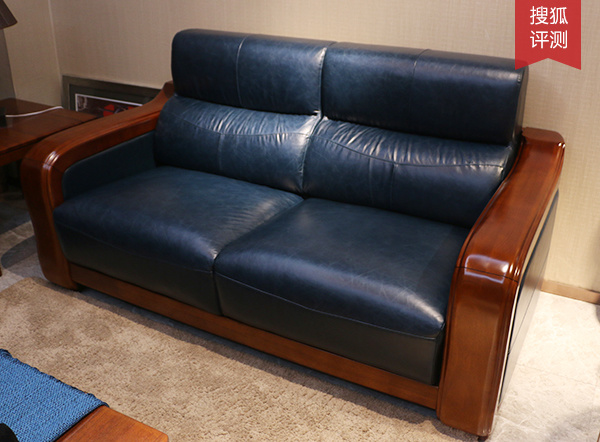稳固简约的实木框架造型，加上饱满高级的优质皮革，将美式家居的优雅大气与现代生活的轻松舒适完美融合。此款沙发精选上等楠木，经久耐用，采用高级皮革和高密度回弹海绵，柔软舒服，尽情躺靠不变形。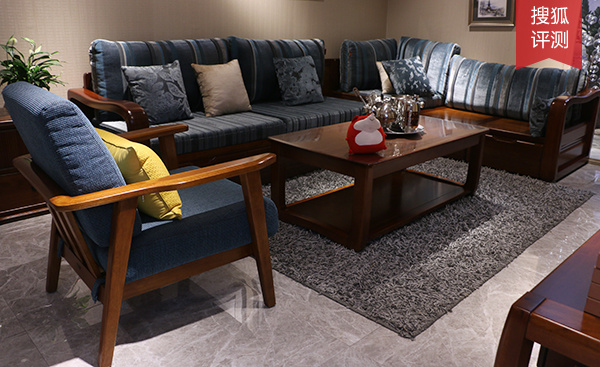优美雅致的线条，清晰丰富的纹理，独特冷冽的色调，使得客厅整体感觉极致清雅。简约不简单的设计，让中小户型也能具有现代美式格调和轻盈自在的美妙意境。此款沙发具备两用功能，冬天可把厚实坐垫放上，柔软舒适，夏天可把坐垫拿开，清凉爽快。沙发设计也暗藏巧思，底部和转角处均设置了大容量储物空间，轻松收纳客厅杂物，存取便利。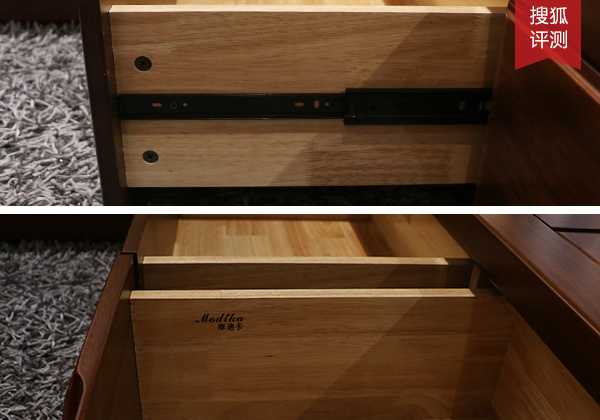沙发抽屉选用优质导轨和五金，确保推拉顺畅，开合静音。抽屉内部做工同样考究，光滑平整没有毛刺，侧边上印有清晰的摩迪卡品牌标识，保真保质，更显档次。此款客厅组合柜适用于中小户型客厅，整体采用上等楠木倾心打造，质感自然，不易开裂变形，兼具实用功能和立体设计，别致耐看。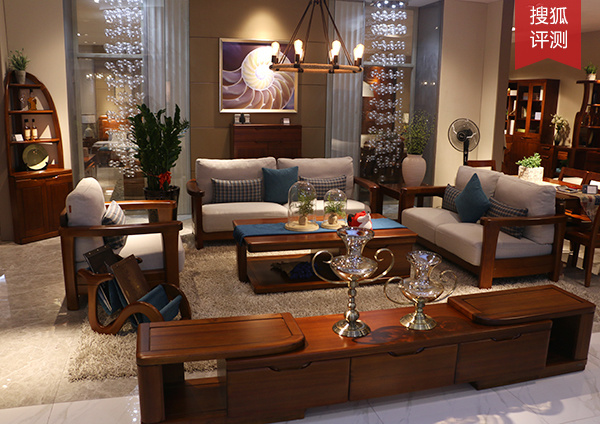别致的布艺实木沙发，方正沉稳的茶几，古典精巧的吊灯，配以相搭的摆饰，营造出温馨简约却不失高贵典雅的客厅空间，让人不自觉想窝在其中，轻享生活。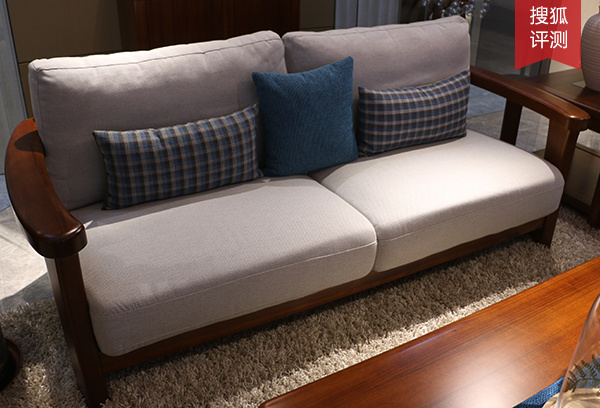素淡的布艺色调增添了清新感，至简的细节设计增加了轻盈感，加上对称的造型，灵动的线条，亲肤的布质，梦之楠沙发自成芳华。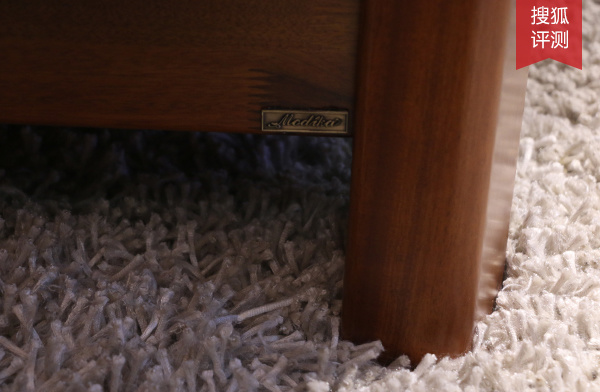梦之楠系列家具产品，整体外观崇尚轻盈简约，不过分张扬，但细节处却能看出其品质不凡，底部的品牌标识复古有个性，与家具巧妙融合，更显档次。此款客厅组合柜与茶几，取自自然，融合自然，运用优质东南亚油楠木精心打造，层次丰富，储物空间多样，无论是整体还是细节都极富设计美感。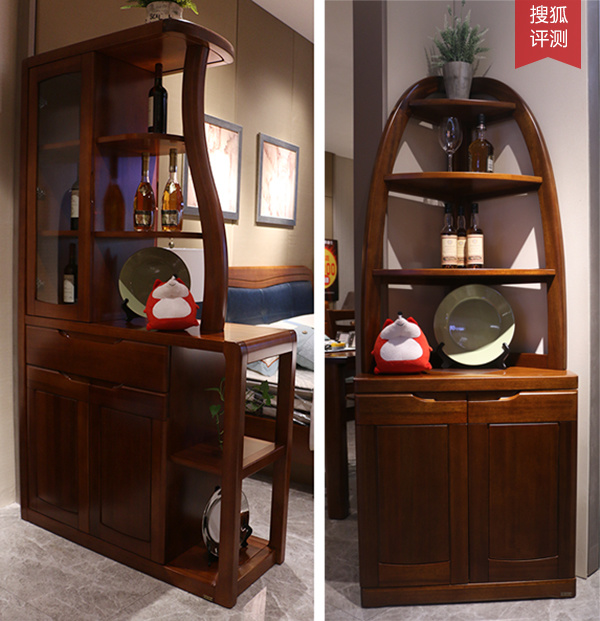梦之楠系列间厅柜，采用多格储物设计和双面装饰设计，占地面积小，层次协调有序，线条婉约优雅，将空间一分为二，给予空间更丰富的表现形式。而图右的装饰柜，同样集功能性与美观性于一体，简约百搭，为家居空间添彩。简约不凡的六斗柜，表面光滑细腻，木纹清晰，能适应多种家装风格，超大柜内空间演绎收纳实力派，给你整洁清爽的轻生活。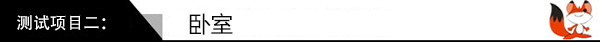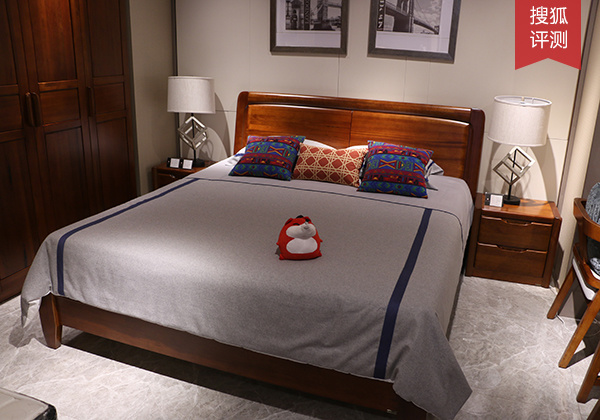此款梦之楠大床，不同于厚重生硬的旧式实木床设计，将美式的优雅与现代的简约注入设计中，展现出全新的实木姿态。此款衣柜简而不凡，稳固高端，采用嵌入式设计，优化利用空间，符合生活美学。实木柜同样品质不凡，材质坚硬，纹理清晰，拉手独特，显示出清雅含蓄的美感。轻简有度的大床，装饰性强的床尾凳，对称分布的床头柜，稳固方正的衣柜，组合出和谐自然、轻盈惬意的卧室空间，配以柔和的灯光，更富有情调。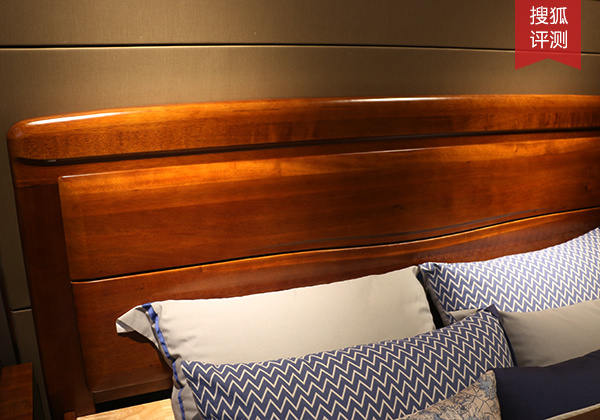床头部分简约质朴，选用进口楠木，手感细腻光滑，纹理丰富清晰，色泽饱满，质感非凡，边角处作圆滑处理，贴心又耐用。此款衣柜整体结构均匀，内部空间却大有文章。衣柜底部设置了带锁抽屉，可放置私密贵重物品，为轻生活增添了一份安心。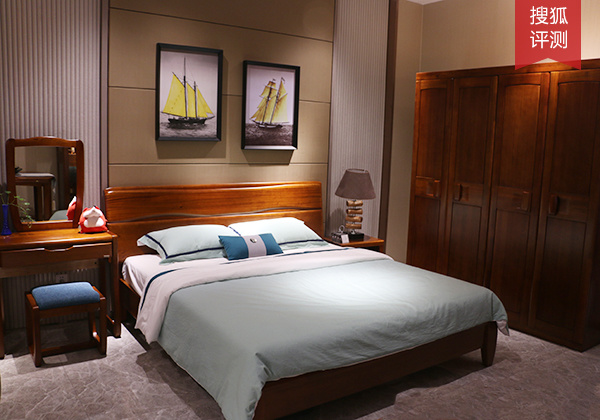梦之楠系列卧室空间特有着一份清雅，木质天然，风韵自然，低调内敛却不失典雅大气，让你纵享舒适惬意的实木卧室轻生活。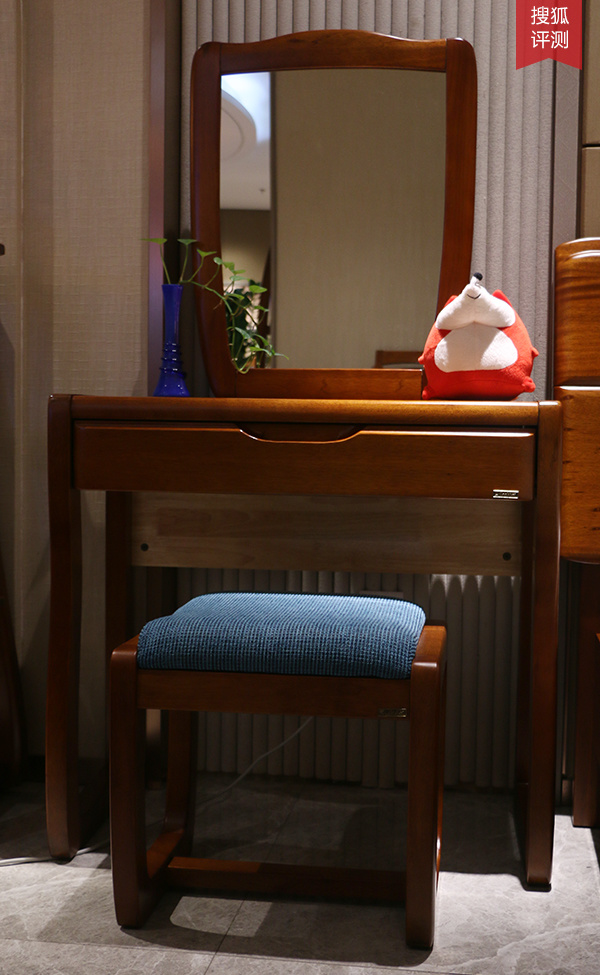梳妆台部分小巧别致，简约实用，工艺出色，平整光滑，进口楠木，稳固耐用，给你与众不同的梳妆体验。梦之楠系列衣柜，注重空间协调，优化柜体设计，科学划分柜内空间。并选用优质楠木，防虫防蛀，板材稳定性强，光泽度强。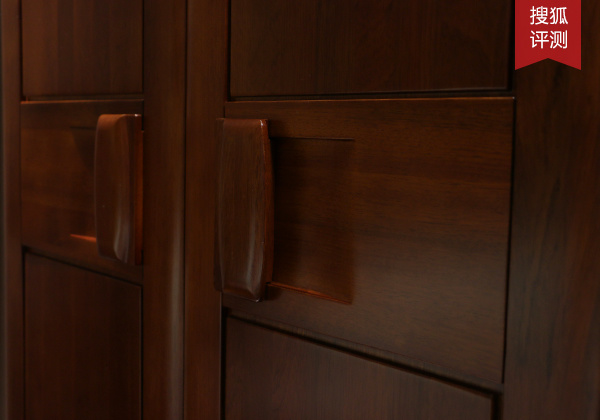此款衣柜用材厚实，材质细腻光滑，纹理清晰，健康环保，品质出众；衣柜拉手参照手部推拉习惯进行设计，圆润独特，简约大方。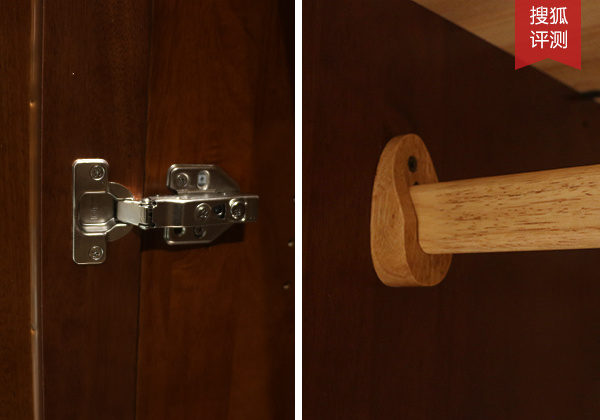衣柜选用优质五金，推拉顺畅，牢固耐用。挂衣杆同样采用实木打造，承重力强，设计合理，给衣物收纳增添更多便捷。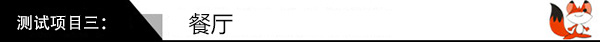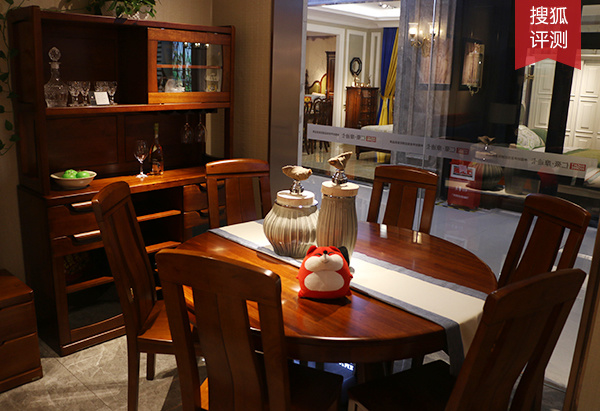梦之楠实木圆桌，造型美观大方，质感典雅醇厚，稳固不摇晃，采用上等楠木，不易开裂，易于清洁。一旁的餐边柜高端大气，层次丰富，打造出品位卓越的餐厅空间。此款餐桌为折叠型餐桌，可根据用餐人数选择折叠或展开，节省空间，方圆随心变换，轻松便捷，实用又耐用。梦之楠系列酒柜，结构对称和谐，外观高端大气，采用进口楠木与优质加厚玻璃打造而成，质量可靠。大小收纳空间充分展示了此款酒柜的实用性，助你品味轻生活。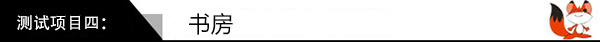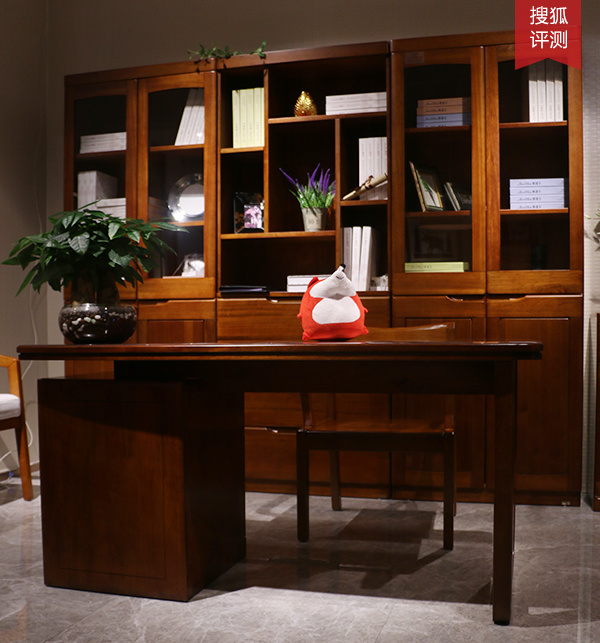梦之楠系列书房，古雅质朴，静谧从容，封闭式、开放式、半开放式储物空间满足不同程度藏书需求，书籍整齐有序排放，书香四溢。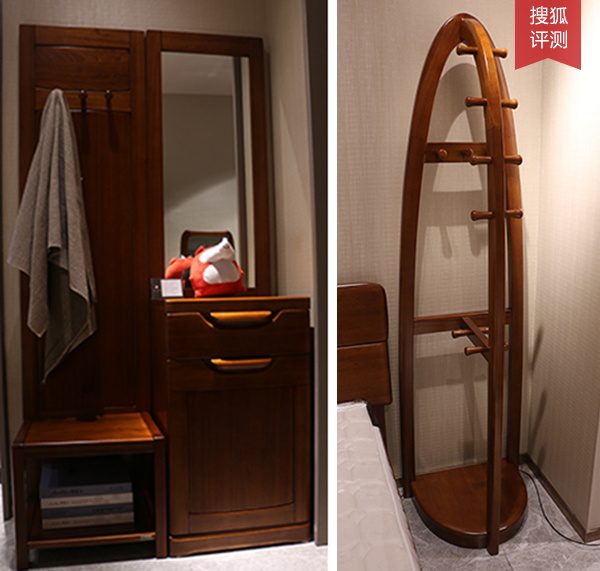光洁的明镜，简约的组合柜，组成实用美观的简易衣帽间。右侧的衣帽架，造型稳固，承重力强，木质坚硬，持久耐用，原木色温馨和谐，更显大方得体。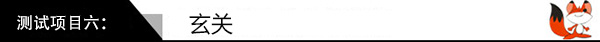此款鞋柜同样坚持选用环保优质楠木，防霉防腐，不易变形，设计简洁直观，功能强大实用，满足大家对自然节约轻生活的追求。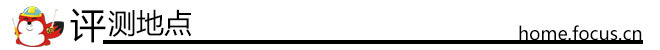评测地点：广州市博皇国际家具博览中心A馆 仁豪·摩迪卡店摩迪卡梦之楠系列产品，采用高级东南亚油楠木精心打造而成，木纹清晰，色泽饱满，质地稳定坚硬，持久耐用。设计简约不凡，去掉不必要的繁冗，构建出简单纯净、自然环保的生活空间，给你梦想中的轻生活。（图文/区文怡）

`声明：本文由入驻焦点开放平台的作者撰写，除焦点官方账号外，观点仅代表作者本人，不代表焦点立场错误信息举报电话： 400-099-0099，邮箱：jubao@vip.sohu.com，或点此进行意见反馈，或点此进行举报投诉。`A B C D E F G H J K L M N P Q R S T W X Y Z
A - B - C - D - E
• A
• 鞍山
• 安庆
• 安阳
• 安顺
• 安康
• 澳门
• B
• 北京
• 保定
• 包头
• 巴彦淖尔
• 本溪
• 蚌埠
• 亳州
• 滨州
• 北海
• 百色
• 巴中
• 毕节
• 保山
• 宝鸡
• 白银
• 巴州
• C
• 承德
• 沧州
• 长治
• 赤峰
• 朝阳
• 长春
• 常州
• 滁州
• 池州
• 长沙
• 常德
• 郴州
• 潮州
• 崇左
• 重庆
• 成都
• 楚雄
• 昌都
• 慈溪
• 常熟
• D
• 大同
• 大连
• 丹东
• 大庆
• 东营
• 德州
• 东莞
• 德阳
• 达州
• 大理
• 德宏
• 定西
• 儋州
• 东平
• E
• 鄂尔多斯
• 鄂州
• 恩施
F - G - H - I - J
• F
• 抚顺
• 阜新
• 阜阳
• 福州
• 抚州
• 佛山
• 防城港
• G
• 赣州
• 广州
• 桂林
• 贵港
• 广元
• 广安
• 贵阳
• 固原
• H
• 邯郸
• 衡水
• 呼和浩特
• 呼伦贝尔
• 葫芦岛
• 哈尔滨
• 黑河
• 淮安
• 杭州
• 湖州
• 合肥
• 淮南
• 淮北
• 黄山
• 菏泽
• 鹤壁
• 黄石
• 黄冈
• 衡阳
• 怀化
• 惠州
• 河源
• 贺州
• 河池
• 海口
• 红河
• 汉中
• 海东
• 怀来
• I
• J
• 晋中
• 锦州
• 吉林
• 鸡西
• 佳木斯
• 嘉兴
• 金华
• 景德镇
• 九江
• 吉安
• 济南
• 济宁
• 焦作
• 荆门
• 荆州
• 江门
• 揭阳
• 金昌
• 酒泉
• 嘉峪关
K - L - M - N - P
• K
• 开封
• 昆明
• 昆山
• L
• 廊坊
• 临汾
• 辽阳
• 连云港
• 丽水
• 六安
• 龙岩
• 莱芜
• 临沂
• 聊城
• 洛阳
• 漯河
• 娄底
• 柳州
• 来宾
• 泸州
• 乐山
• 六盘水
• 丽江
• 临沧
• 拉萨
• 林芝
• 兰州
• 陇南
• M
• 牡丹江
• 马鞍山
• 茂名
• 梅州
• 绵阳
• 眉山
• N
• 南京
• 南通
• 宁波
• 南平
• 宁德
• 南昌
• 南阳
• 南宁
• 内江
• 南充
• P
• 盘锦
• 莆田
• 平顶山
• 濮阳
• 攀枝花
• 普洱
• 平凉
Q - R - S - T - W
• Q
• 秦皇岛
• 齐齐哈尔
• 衢州
• 泉州
• 青岛
• 清远
• 钦州
• 黔南
• 曲靖
• 庆阳
• R
• 日照
• 日喀则
• S
• 石家庄
• 沈阳
• 双鸭山
• 绥化
• 上海
• 苏州
• 宿迁
• 绍兴
• 宿州
• 三明
• 上饶
• 三门峡
• 商丘
• 十堰
• 随州
• 邵阳
• 韶关
• 深圳
• 汕头
• 汕尾
• 三亚
• 三沙
• 遂宁
• 山南
• 商洛
• 石嘴山
• T
• 天津
• 唐山
• 太原
• 通辽
• 铁岭
• 泰州
• 台州
• 铜陵
• 泰安
• 铜仁
• 铜川
• 天水
• 天门
• W
• 乌海
• 乌兰察布
• 无锡
• 温州
• 芜湖
• 潍坊
• 威海
• 武汉
• 梧州
• 渭南
• 武威
• 吴忠
• 乌鲁木齐
X - Y - Z
• X
• 邢台
• 徐州
• 宣城
• 厦门
• 新乡
• 许昌
• 信阳
• 襄阳
• 孝感
• 咸宁
• 湘潭
• 湘西
• 西双版纳
• 西安
• 咸阳
• 西宁
• 仙桃
• 西昌
• Y
• 运城
• 营口
• 盐城
• 扬州
• 鹰潭
• 宜春
• 烟台
• 宜昌
• 岳阳
• 益阳
• 永州
• 阳江
• 云浮
• 玉林
• 宜宾
• 雅安
• 玉溪
• 延安
• 榆林
• 银川
• Z
• 张家口
• 镇江
• 舟山
• 漳州
• 淄博
• 枣庄
• 郑州
• 周口
• 驻马店
• 株洲
• 张家界
• 珠海
• 湛江
• 肇庆
• 中山
• 自贡
• 资阳
• 遵义
• 昭通
• 张掖
• 中卫

1室1厅1厨1卫1阳台

1
2
3
4
5

0
1
2

1

1

0
1
2
3报名成功，资料已提交审核A B C D E F G H J K L M N P Q R S T W X Y Z
A - B - C - D - E
• A
• 鞍山
• 安庆
• 安阳
• 安顺
• 安康
• 澳门
• B
• 北京
• 保定
• 包头
• 巴彦淖尔
• 本溪
• 蚌埠
• 亳州
• 滨州
• 北海
• 百色
• 巴中
• 毕节
• 保山
• 宝鸡
• 白银
• 巴州
• C
• 承德
• 沧州
• 长治
• 赤峰
• 朝阳
• 长春
• 常州
• 滁州
• 池州
• 长沙
• 常德
• 郴州
• 潮州
• 崇左
• 重庆
• 成都
• 楚雄
• 昌都
• 慈溪
• 常熟
• D
• 大同
• 大连
• 丹东
• 大庆
• 东营
• 德州
• 东莞
• 德阳
• 达州
• 大理
• 德宏
• 定西
• 儋州
• 东平
• E
• 鄂尔多斯
• 鄂州
• 恩施
F - G - H - I - J
• F
• 抚顺
• 阜新
• 阜阳
• 福州
• 抚州
• 佛山
• 防城港
• G
• 赣州
• 广州
• 桂林
• 贵港
• 广元
• 广安
• 贵阳
• 固原
• H
• 邯郸
• 衡水
• 呼和浩特
• 呼伦贝尔
• 葫芦岛
• 哈尔滨
• 黑河
• 淮安
• 杭州
• 湖州
• 合肥
• 淮南
• 淮北
• 黄山
• 菏泽
• 鹤壁
• 黄石
• 黄冈
• 衡阳
• 怀化
• 惠州
• 河源
• 贺州
• 河池
• 海口
• 红河
• 汉中
• 海东
• 怀来
• I
• J
• 晋中
• 锦州
• 吉林
• 鸡西
• 佳木斯
• 嘉兴
• 金华
• 景德镇
• 九江
• 吉安
• 济南
• 济宁
• 焦作
• 荆门
• 荆州
• 江门
• 揭阳
• 金昌
• 酒泉
• 嘉峪关
K - L - M - N - P
• K
• 开封
• 昆明
• 昆山
• L
• 廊坊
• 临汾
• 辽阳
• 连云港
• 丽水
• 六安
• 龙岩
• 莱芜
• 临沂
• 聊城
• 洛阳
• 漯河
• 娄底
• 柳州
• 来宾
• 泸州
• 乐山
• 六盘水
• 丽江
• 临沧
• 拉萨
• 林芝
• 兰州
• 陇南
• M
• 牡丹江
• 马鞍山
• 茂名
• 梅州
• 绵阳
• 眉山
• N
• 南京
• 南通
• 宁波
• 南平
• 宁德
• 南昌
• 南阳
• 南宁
• 内江
• 南充
• P
• 盘锦
• 莆田
• 平顶山
• 濮阳
• 攀枝花
• 普洱
• 平凉
Q - R - S - T - W
• Q
• 秦皇岛
• 齐齐哈尔
• 衢州
• 泉州
• 青岛
• 清远
• 钦州
• 黔南
• 曲靖
• 庆阳
• R
• 日照
• 日喀则
• S
• 石家庄
• 沈阳
• 双鸭山
• 绥化
• 上海
• 苏州
• 宿迁
• 绍兴
• 宿州
• 三明
• 上饶
• 三门峡
• 商丘
• 十堰
• 随州
• 邵阳
• 韶关
• 深圳
• 汕头
• 汕尾
• 三亚
• 三沙
• 遂宁
• 山南
• 商洛
• 石嘴山
• T
• 天津
• 唐山
• 太原
• 通辽
• 铁岭
• 泰州
• 台州
• 铜陵
• 泰安
• 铜仁
• 铜川
• 天水
• 天门
• W
• 乌海
• 乌兰察布
• 无锡
• 温州
• 芜湖
• 潍坊
• 威海
• 武汉
• 梧州
• 渭南
• 武威
• 吴忠
• 乌鲁木齐
X - Y - Z
• X
• 邢台
• 徐州
• 宣城
• 厦门
• 新乡
• 许昌
• 信阳
• 襄阳
• 孝感
• 咸宁
• 湘潭
• 湘西
• 西双版纳
• 西安
• 咸阳
• 西宁
• 仙桃
• 西昌
• Y
• 运城
• 营口
• 盐城
• 扬州
• 鹰潭
• 宜春
• 烟台
• 宜昌
• 岳阳
• 益阳
• 永州
• 阳江
• 云浮
• 玉林
• 宜宾
• 雅安
• 玉溪
• 延安
• 榆林
• 银川
• Z
• 张家口
• 镇江
• 舟山
• 漳州
• 淄博
• 枣庄
• 郑州
• 周口
• 驻马店
• 株洲
• 张家界
• 珠海
• 湛江
• 肇庆
• 中山
• 自贡
• 资阳
• 遵义
• 昭通
• 张掖
• 中卫• 手机• 分享
• 设计
免费设计
• 计算器
装修计算器
• 入驻
合作入驻
• 联系
联系我们
• 置顶
返回顶部